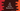# 3 ways in C# to find the nth number of the Fibonacci series## How to find the nth number of the Fibonacci series in C#:

In this post, we will learn how to find the nth number of the Fibonacci series. This program will take the value of n as an input from the user and print the nth number of the Fibonacci series.

The Fibonacci series is an infinite series of numbers, it starts with 0, 1 and all other values are equal to the sum of last two numbers.

0, 1, 1, 2, 3, 5, 8, 13, 21, 34 are the first 10 numbers of the Fibonacci series. Each number is equal to the sum of previous two numbers.

### Method 1: Iterative approach by using a for loop:

In this method, we will write a program that will use a for loop to find the nth number of the Fibonacci series. On each iteration, the loop will find the sum of last two numbers to find the current number.

``````using System;

namespace Program
{
class Program
{
static int GetNthFibonacci(int n)
{
if (n <= 1) return 0;

int First = 0, Second = 1, Current;

for (int i = 3; i < n; i++)
{
Current = First + Second;
First = Second;
Second = Current;
}

return First + Second;
}
static void Main(string[] args)
{
int n;

Console.WriteLine("Enter the value of n: ");

Console.WriteLine("Fibonacci number at position {0}: {1}", n, GetNthFibonacci(n));
}
}
}``````

Here,

• GetNthFibonacci method is used to get the nth fibonacci number. It takes the value of n as its parameter and returns the nth Fibonacci number.
• The for loop is used to update the First and Second values. These values are 0 and 1 at the start of the program, i.e. these are the initial values of the series.
• Once the loop ends, it returns the sum of First and Second, which is the required nth value.

If you run this program, it will print output as like below:

``````Enter the value of n:
4
Fibonacci number at position 4: 3

Enter the value of n:
6
Fibonacci number at position 6: 5

Enter the value of n:
10
Fibonacci number at position 10: 34``````

### Method 2: By using a while loop:

We can also use a while loop to write the same program.

``````using System;

namespace Program
{
class Program
{
static int GetNthFibonacci(int n)
{
if (n <= 1) return 0;

int First = 0, Second = 1, Current, i = 3;

while (i < n)
{
Current = First + Second;
First = Second;
Second = Current;
i++;
}

return First + Second;
}
static void Main(string[] args)
{
int n;

Console.WriteLine("Enter the value of n: ");

Console.WriteLine("Fibonacci number at position {0}: {1}", n, GetNthFibonacci(n));
}
}
}``````

It is similar to the above program. For while loop, we have to initialize the value of i before the loop starts and at the end of each iteration of the loop, we are incrementing the value of i by 1.

It will give similar result.

### Method 3: Recursive way to find the nth Fibonacci series number:

This is another way to find the nth Fibonacci series number. A recursive method calls itself again and again to find the result. We will define the base cases where to stop the recursive call.

``````using System;

namespace Program
{
class Program
{
static int GetNthFibonacci(int n)
{
if (n == 1) return 0;
if (n == 2) return 1;

return GetNthFibonacci(n - 1) + GetNthFibonacci(n - 2);
}
static void Main(string[] args)
{
int n;

Console.WriteLine("Enter the value of n: ");